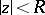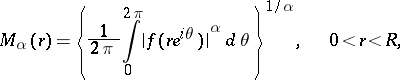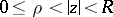# Hardy theorem

(diff) ← Older revision | Latest revision (diff) | Newer revision → (diff)

in the theory of functions of a complex variable

Ifis a regular analytic function in the disc,is a positive number, and ifis the average value, thenis a non-decreasing function ofthat is logarithmically convex relative to(cf. Convexity, logarithmic). The theorem was established by G.H. Hardy .

The assertion on the logarithmic convexity remains valid for a functionthat is regular in an annulus(see ).

Hardy's theorem generalizes to subharmonic functions (cf. Subharmonic function) in a ball of,(see also ).

How to Cite This Entry:
Hardy theorem. Encyclopedia of Mathematics. URL: http://encyclopediaofmath.org/index.php?title=Hardy_theorem&oldid=16176
This article was adapted from an original article by E.D. Solomentsev (originator), which appeared in Encyclopedia of Mathematics - ISBN 1402006098. See original article